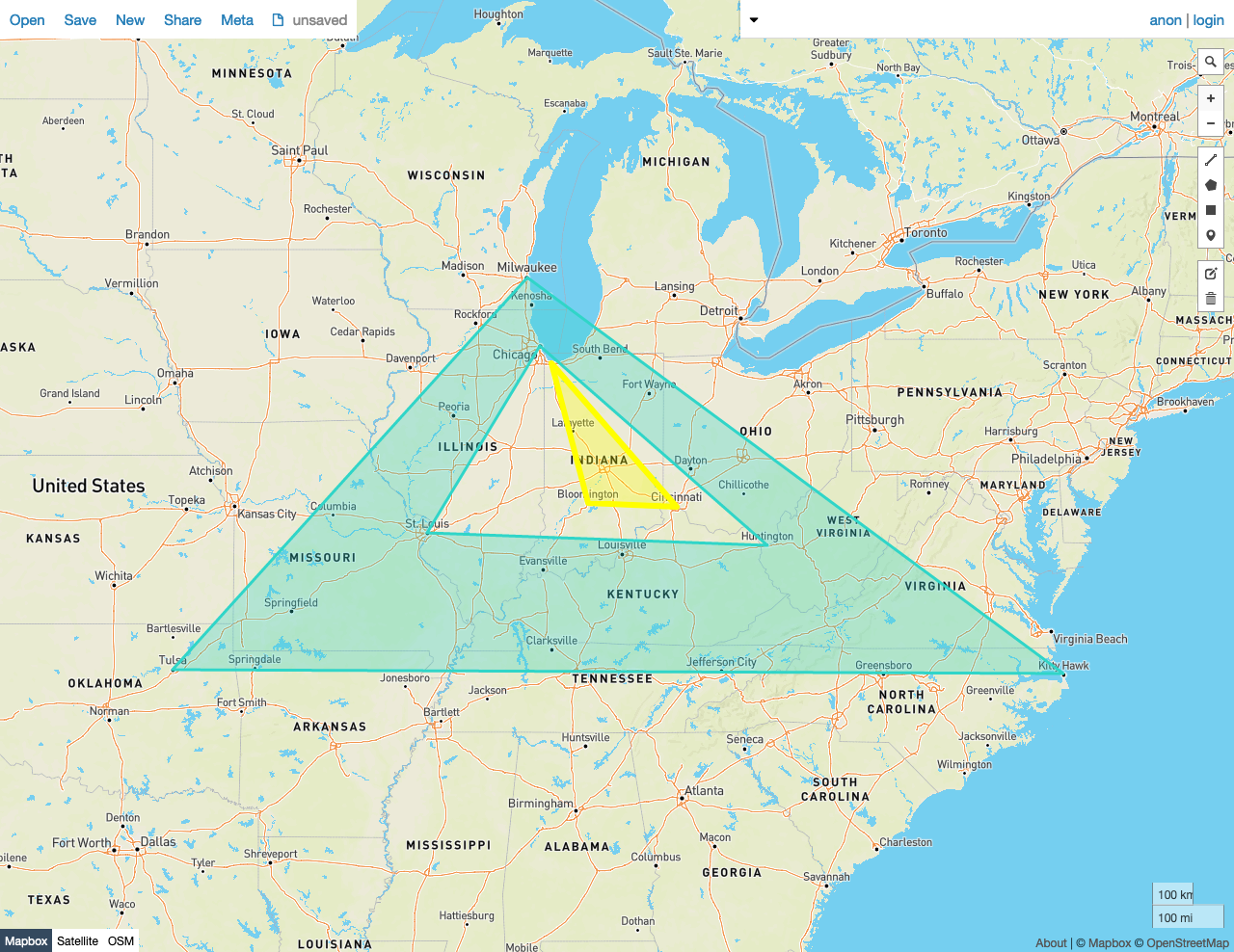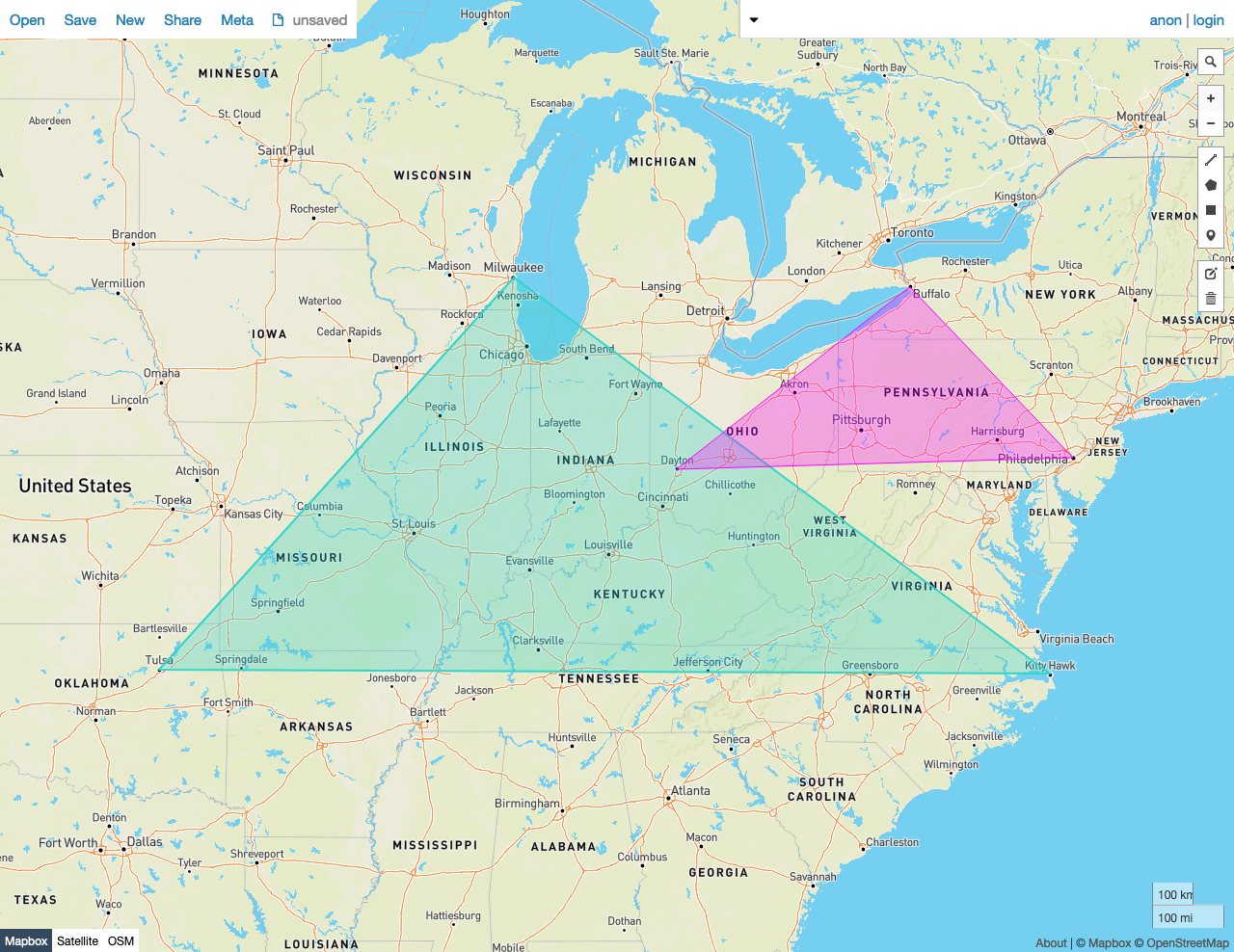On this pageGiven two shapes A and B, `ST_Disjoint(A, B)` returns `true` if the shapes do not share any of the same space -- that is, if no point in the set that comprises A is also a member of the set of points that make up B.

`ST_Disjoint` works on the following spatial data types:

Note:

`ST_Disjoint` does not make use of spatial indexes.

Note:

This function is the inverse of `ST_Intersects`.

## Examples

Note:

The screenshots in these examples were generated using geojson.io, but they are designed to showcase the shapes, not the map. Representing `GEOMETRY` data in GeoJSON can lead to unexpected results if using geometries with SRIDs other than 4326 (as shown below).

### True

In this example, `ST_Disjoint` returns `true` because:

• No Point in the set that comprises Polygon A is also a member of the set of points that make up Polygon B.
``````SELECT st_disjoint(st_geomfromtext('SRID=4326;POLYGON((-87.906471 43.038902, -95.992775 36.153980, -75.704722 36.076944, -87.906471 43.038902), (-87.623177 41.881832, -90.199402 38.627003, -82.446732 38.413651, -87.623177 41.881832))'), st_geomfromtext('SRID=4326;POLYGON((-87.356934 41.595161, -84.512016 39.103119, -86.529167 39.162222, -87.356934 41.595161))'));
``````
``````  st_disjoint
---------------
true

(1 row)
``````### False

In this example, `ST_Disjoint` returns `false` because:

• Many Points in the set that comprises Polygon A are also members of the set of points that make up Polygon B.
``````SELECT st_disjoint(st_geomfromtext('SRID=4326;POLYGON((-87.906471 43.038902, -95.992775 36.153980, -75.704722 36.076944, -87.906471 43.038902))'), st_geomfromtext('SRID=4326;POLYGON((-84.191605 39.758949, -75.165222 39.952583, -78.878738 42.880230, -84.191605 39.758949))'));
``````
``````  st_disjoint
---------------
false
(1 row)
``````